Excel
STATISTICAL
Master

The Normal Distribution

Clear and Complete - WITH LOTS OF SOLVED PROBLEMS

# Normal Distribution

Lots of Worked-Out, Easy-To-Understand, Graduate-Level Problems  --->
( Scroll Down and Take a Look ! )

Introduction

The Normal distribution is one of the fundamental building blocks of statistics. The Normal distribution is also called the Gaussian distribution within the scientific community. Many measurements and physical phenomena can be approximated with the Normal distribution. The Normal Distribution has applications in many areas of business. For example, portfolio returns and human performance often follow a Normal Distribution.

## Basic Description of Normal Distribution

The shape of the Normal distribution resembles a bell so it is sometimes called the "bell curve." The Normal curve is symmetric about its mean in the middle, and its tails on either side extend to infinity. The Normal distribution is a continuous function, not a discrete function, This means that any value can be graphed somewhere on a Normal curve. Discrete functions only map specific values, such as whole numbers.

If a large enough random sample is taken (n>30) from an unknown population, the mean of the population of any underlying distribution can be estimated on the Normal curve. This is a result of statistics' most important theorem, the Central Limit Theorem, and will be discussed in this section.

The Normal Distribution is actually a family of Normal Distributions, each Normal Distribution having a unique combination of mean and standard deviation. The basic Normal Distribution is called the Standard Normal Distribution and has a standard deviation equal to 1 and a mean equal to 0. Normal Probability - A Very Basic Definition

## Mapping the Normal Distribution

Any Normal distribution can be completely mapped if only the following two parameters are known:

1) Mean - µ - the Greek symbol "mu"

2) Standard deviation - σ - the Greek symbol "sigma"

If only the mean and standard deviation of a Normal distribution are known, then every point of the Normal curve can be mapped.

*************************************************************

A Standardized Normal Curve is a Normal curve

that has mean = 0 and standard deviation = 1

*************************************************************

The "68 - 95 - 99.7%" Rule for the Normal distribution states that:

68% of all observations lie within 1 standard deviation of the mean,
within the range of µ +/- s

95% of all observations lie within 2 standard deviations of the mean,
within the range of µ +/- 2s

99.7% of all observations lie within 3 standard deviations of the mean,
within the range of µ +/- 3s

50% of the Normal Distribution lies within 0.6745 standard deviations of the mean.

## Standardized Normal Curve

The Standard Normal Distribution is the normal distribution with a mean of zero and the standard deviation equal to one.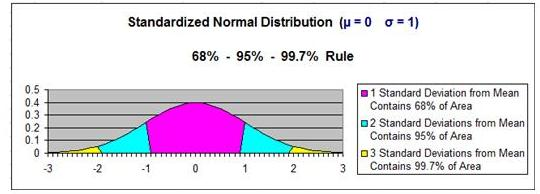## Non-Standardized Normal Curve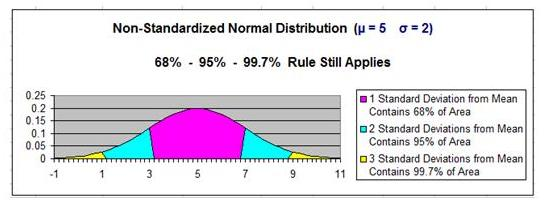## Normal Distribution Probability Density Function

The Probability Density Function calculates the probability that distributed variable X will equal a specific value of X = x. The Probability Density Function of the Normal curve is what is often referred to as the Bell Curve. This is the Normal curve and can be characterized by the following:

1. The “Bell Curve” is symmetrical about its mean.
2. The mode and the median both equal the mean.
3. The inflection points of the curve occur one standard deviation above and below the mean. At the inflection points, the second derivative of x with respect to y changes sign.
4. Nearly all of the area under the Normal Distribution curve (99.7% of the total area) occurs within 3 standard deviations from the mean.
5. The area under the curve = 1
6. This curve extends to +/- ∞.
7. The curve is asymptotic to the x axis, meaning that the curve gets closer and closer to the horizontal axis but never quite reaches it.

The Probability Density Function is given by:

f(x) = { 1 / [σ SQRT(2π)] } * exp [ - { (x - µ)2 / 2σ2 } ]

The Probability Density Function is expressed with a lowercase f(x) but the Cumulative Distribution Function (discussed below) is usually expressed with an uppercase F(x)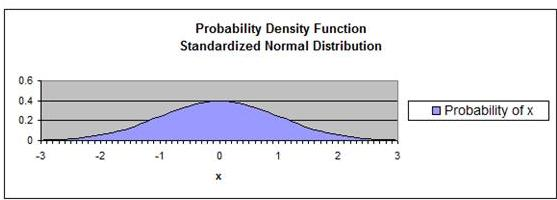## Normal Distribution Cumulative Distribution Function

The Cumulative Distribution Function (CDF) of a probability distribution, evaluated at a number (X = x), is the probability that random variable (capital X) will be less than or equal to x. The CDF varies between 0 (0% of the event occurring at x = -∞) and 1 (100% of the event occurring at x = +∞). The CDF will represent the percentage of area under the curve to the left of X = x.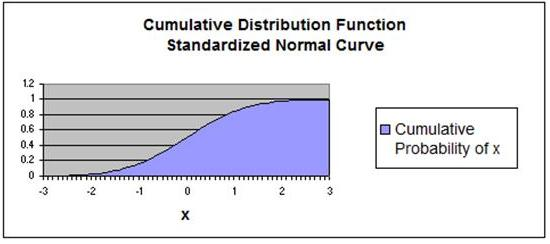## The Central Limit Theorem

The Central Limit Theorem, one of Statistics’ most important underlying theorems, states the following: Random sample data can be plotted on a Normal curve to estimate a population's mean no matter how the population is distributed, as long as sample size is large (n>30).

No matter how the population is distributed, the sampling distribution of the mean approaches the Normal curve as sample size becomes large (at least 30).

The Central Limit Theorem is also the underlying basis that the Normal Distribution:

Approximates the Binomial Distribution when Binomial Distribution parameter p is not too close to 1 or 0 and np > 10.

Approximates the Poisson Distribution when Poisson Distribution parameter λ is large.
.

## Normality Tests

Normality tests check a given set of data for similarity to the Normal Distribution. The Null Hypothesis is that the data set is similar to the Normal Distribution, therefore a a sufficiently small p value indicates non-normal data.

- Kolmogorov-Smirnov test
- Lilliefors test
- Anderson-Darling test
- Ryan-Jointer test
- Jarqu-Bera test

## Z Score

The Z score is the number of standard deviations from the mean to a point on the x axis which corresponds to a specified percentage of area under the Normal curve from the mean to that x-axis point.

For example, 68.26% of the total area under the Normal curve falls within 1 standard deviation of the mean. That means that 34.13% of the total area under the Normal curve lies within 1 standard deviation above the mean and 34.13% of the total area under the Normal curve lies within 1 standard deviation below the mean. This translates to the following:

The point on the x axis which corresponds to a Z score of 1.00 is 1.00 standard deviations from the mean. 34.13% of the total area under the Normal curve lies between this point on the x axis and the mean.

If, for example, you are trying to determine how many standard deviations above and below the mean will contain 95% of the total area under the Normal curve, you would locate the Z score that corresponds within the point containing 47.5% of the total area under the curve above the mean.

The Z score = [ ( Value – Mean) / Standard deviation ] = [ (x - µ ) / µ ]

The distribution of z values is the same as the Standard Normal Distribution, which has a mean of zero and a standard deviation of 1. As stated above, a Z score of 1.00 corresponds to the point the is one standard deviation from the mean. 34.13% of the total area under the Normal curve lies one standard deviation above and also one standard deviation below the mean.

If tools such as Excel are not available, then problems involving the Normal Distribution are often solved using tables. Take a look at the problems in this section to see detailed examples.

The two-tailed Z score tells how many standard deviations from the mean are required to contain a specified percentage of the area under the Normal Curve.

In the case of this two-tailed, 95% Z score, 95% of the total area under the Normal curve is contained within 1.96 standard deviations from the mean in either direction of the mean.

For this two-tailed test, the remaining 5% of the area under the Normal Curve is split between the 2 outer tails (hence the name - "two-tailed). Each outer tail holds 2.5% of the total area under the Normal Curve. This leaves 47.5% of the total area between the outer tail and the mean on each side of the mean.

The Z score of 1.96 corresponds to this remaining 47.5% of the total area between the mean and each outer tail containing 2.5% of the total area.

Before Z tables can be used to solved statistical problems, it must be known for certain that the population from which samples are taken is Normally distributed. If the underlying population is not normally distributed, the resulting answer may be incorrect.

## One-Tailed Normal Curve - Right Tail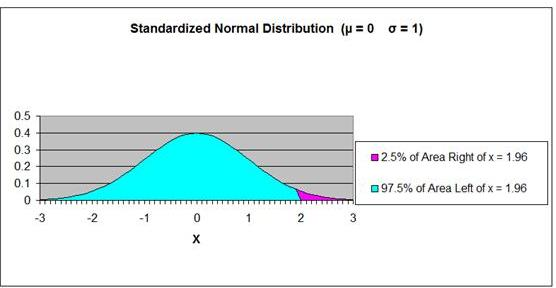## One-Tailed Normal Curve - Left Tail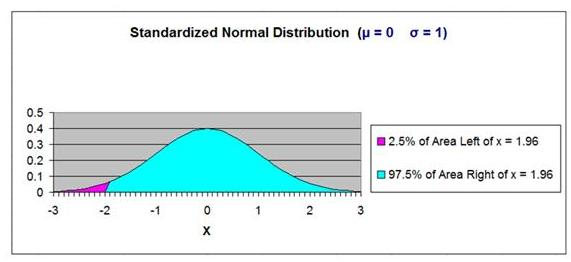## Using the Binomial Distribution to Estimate the Normal Distribution

The Normal Distribution can be estimated using the Binomial Distribution if n is large and p and q are not too close to 0. If variable X is Binomially distributed and has parameters n (number of trials) and p (probability of success on each trial), for large values of n the distribution of X is approximately Normal. The mean and variance of the approximately Normal distribution of X are np and np(1-p). These are the same as the mean and variance of Binomial distribution that has parameters n and p.

The Normal approximation does not work well for small values of n. The Normal approximation should only be used if np>10 and np(1-p)>10.

For example, if n = 1000 and p = 0.4, then np = 400 and np(1-p) = 240.

Since np>10 and np(1-p)>10, then:

the Normal and Binomial Probability Density Functions should be very close for various values of the count variable X. This is demonstrated in the table below:

 Normal (X, µ, σ2) = n p np np(1-p) X Binomial (X, n, p) Normal (X, np, np(1-p) ) 1000 0.4 400 240 385 0.0162 0.0161 400 0.0257 0.0258 415 0.0160 0.0161

## Using the Normal Distribution to Estimate the Poisson Distribution

The Normal Distribution is approximately equal to the Poisson Distribution when Poisson parameter λ is large. The Normal Distribution which approximates the Poisson Distribution has the parameters µ = σ2  = λ.

 Normal (X, µ, σ2) = λ µ σ X Poisson (X, λ) Normal (X, λ, λ) 0.4 400 240 385 0.0153 0.0151 400 0.0199 0.0199 415 0.0148 0.0151

Problem 1

Problem 1: Using the Normal Distribution to Determine that Probability of Daily Production Level Will Be Below a Certain Point

A production machine has normally distributed daily output in units. The average daily output is 4000 and daily output standard deviation is 500. What is the probability that the production of one random day will be below 3580?

Problem Parameter Outline

Population Mean = µ = "mu" = 4,000

Population Standard Deviation = σ = "sigma" =  500

x = 3,580

Probability x ≤ 3,580 = ?

Sales are Normally distributed

Normal curve is not standardized (µ ≠ 0, σ ≠ 1)

Problem Solving Steps

We know that Production data is Normally distributed and can therefore be mapped on the Normal curve.

We are trying to determine the probability that Production Output will be below 3,580 units on any given day.

This probability corresponds to the percentage of area under the Normal curve that has an x value below (to the left of) 3,580.

If we know how many standard deviations x = 3,580 is from the mean (µ = 4,000), we can use the Z Score Chart what percentage of total area under the Normal curve is between x = 3,580 and the mean.

Number of standard deviations x = 3,580 is from the mean  = Zx=3,580

z = ( x - µ ) / σ

Zx=3,580 = (3,580  - 4,000) / 500 = -0.84

The point x = 3,580 is 0.84 standard deviations from the mean
µ = 4,000. Because Zx=3,580 = -0.84 has a negative sign, that point is to the left of the mean.

The Z Score Chart below shows that 30% (0.30) of the total area under the Normal curve will be between the mean and a point that 0.84 standard deviations from the mean.

If 30% of the total area under the Normal curve is between x = 3,580 and the mean µ = 4,000, then 20% of the total area under the Normal curve must be outside this x value in the outer left tail of the Normal curve, as is shown in the graph of the Normal curve below.

We obtain this percentage by subtracting 0.30 (30%) from 0.5.  The mean divides the Normal curve in half and each half on either side of the mean contains 50% of the total area under the Normal curve.

This 20% of the area under the Normal curve that is to the left of x = 3,580 corresponds to the answer that that there is 20% probability that the Production Level will be less than 3,580 on any given day

Answer: There is a 20% probability that the Production Level will fall below 3,580 units on any given day.

Z Score Chart

Z Score at x (Inner Numbers - Yellow)
vs.
Area Under Normal Curve
Between Mean (µ) and x  (Outer Numbers - Green)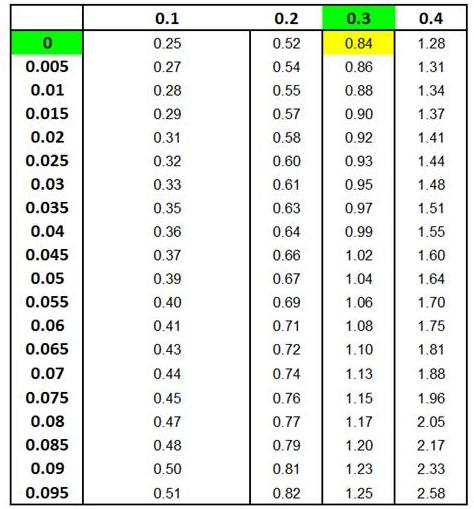Answer: There is a 20% probability that the Production Level will fall below 3,580 units on any given day.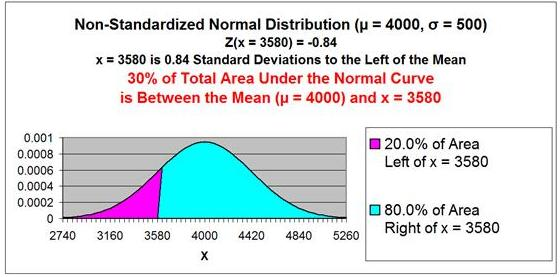This same problem above is solved in the Excel Statistical Master with only ONE Excel formula (and not having to looking anything up on a Z Chart). If you found your statistics book confusing, You'll really like the Excel Statistical Master. Everything is explained in simple, step-by-step frameworks.

Problem 2

Problem 2: Using the Normal Distribution to Determine the Probability that Daily Sales Will Be Within a Certain Range

A salesperson has a mean daily sales of 51 units with a standard deviation of 5 units. What percentage of daily sales can be expected to be between 48 units AND 53 units? Weight is normally distributed for daily sales.

Problem Parameter Outline

Population Mean = µ = "mu" = 51 units

Population Standard Deviation = σ = "sigma" = 5 units

48 units ≤ x ≤ 53 units

Probability that 43 ≤ x ≤ 53 = ?

units are Normally distributed

Normal curve is not standardized (µ ≠ 0, σ ≠ 1)

Problem Solving Steps

We know that Daily Sales data is Normally distributed and can therefore be mapped on the Normal curve.

We are trying to determine the probability that Daily Sales will be between 48 and 53 on any given day.

This probability corresponds to the percentage of area under the Normal curve that has an x value of 48 on the lower (left) side and an x value of 53 on the upper (right) side.

If we know how many standard deviations x = 48 and x = 53 each are from the mean (µ = 50), we can use the Z Score Charts to find what percentage of total area under the Normal curve is between x = 48 and x = 53.

*****************************************************************

Number of standard deviations x = 48 is from the mean  = Zx=48

z = ( x - µ ) / σ

Zx=48 = (48  - 50) / 5 = -0.60

The point x = 48 is 0.60 standard deviations from the mean µ = 50. Because Zx=48 = -0.60 has a negative sign, that point is to the left of the mean.

The 2nd Z Score Chart below shows that 22.5% (0.225) of the total area under the Normal curve will be between the mean and a point (x = 48) that 0.60 standard deviations from the mean.

If 22.5% of the total area under the Normal curve is between x = 48 and the mean (x = 50), 27.5% of the total area under the Normal curve must exist in the outer left tail to the left of x = 48. This is illustrated in the 2nd graph below.

*****************************************************************

Number of standard deviations x = 53 is from the mean  = Zx=53

z = ( x - µ ) / σ

Zx=53 = (53  - 50) / 5 = 0.40

The point x = 53 is 0.40 standard deviations from the mean
µ = 50. Because Zx=53 = 0.40 has a positive sign, that point is to the right of the mean.

The 1st Z Score Chart below shows that 15.5% (0.115) of the total area under the Normal curve will be between the mean and a point that 0.40 standard deviations from the mean. This is also shown in the 1st graph below.

if 15.5% of the total area under the Normal curve exists between the point x = 53 and the mean, then 65.5% of the total area under the Normal curve is to the left of the point x = 53. This is also illustrated in the 1st graph shown below.

*****************************************************************

The total area under the Normal curve between x = 48 and x = 53 equals the area to the left of X = 53 (65.5%) MINUS the area to the left x = 48 (27.5%).

65.5% - 27.5% = 38%

38% of the total area under the Normal curve exists between x = 48 and x = 53. This is shown in the 3rd graph below.

Answer: There is a 38% probability that Daily Sales will fall between 48 units and 53 units on any given day.

Z Score Chart

Z Score at x (Inner Numbers - Yellow)
vs.
Area Under Normal Curve
Between Mean (µ) and x  (Outer Numbers - Green)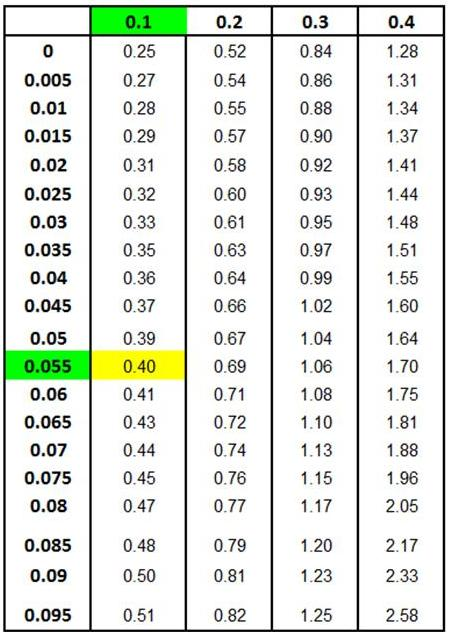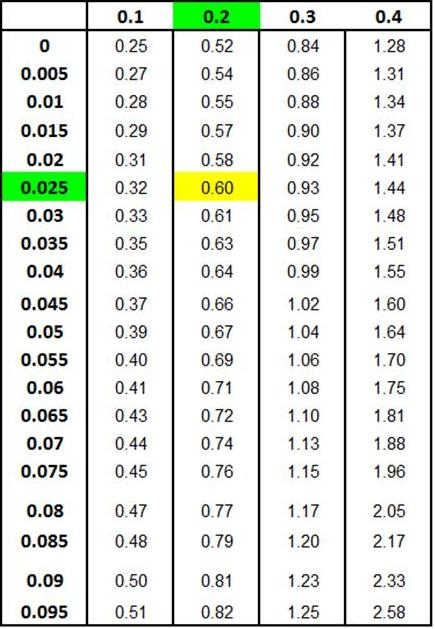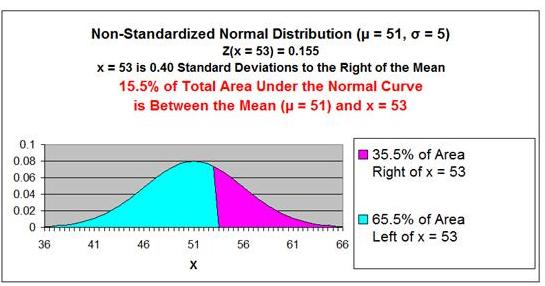MINUS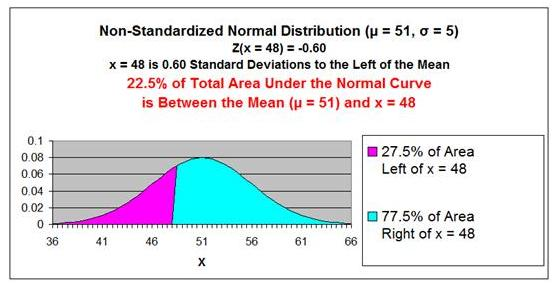EQUALS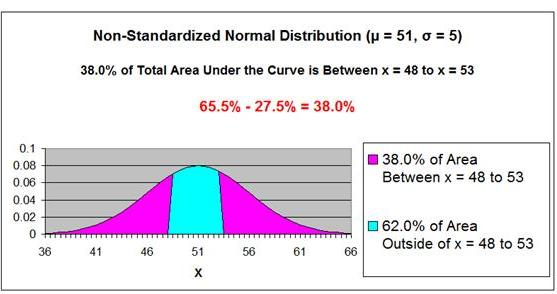Answer: There is a 38% probability that Daily Sales will fall between 48 units and 53 units on any given day.

This same problem is solved in the Excel Statistical Master with only 1 Quick Excel formula (and not haveing to look up ANYTHING on a Z Chart). The Excel Statistical Master teaches you everything in step-by-step frameworks. You'll never have to memorize any complicated statisical theory.

Problem 3

Problem 3: Using the Normal Distribution to Determine the Lower 10% Limit of Delivery Times

A pizza deliveryman's delivery time is normally distributed with a mean of 20 minutes and a standard deviation of 4 minutes. What delivery time will be beaten by only 10% of all deliveries?

Problem Parameter Outline

Population Mean = µ = "mu" = 20 minute

Population Standard Deviation = σ = "sigma" = 4 minutes

x = ?

Probability that (delivery time ≤ x) = 10% = 0.10

Delivery time is Normally distributed

Normal curve is not standardized (µ ≠ 0, σ ≠ 1)

Problem Solving Steps

We know that Delivery Time data is Normally distributed and can therefore be mapped on the Normal curve.

We are trying to determine Delivery Time will be lower than 90% of all Delivery Times.

This probability corresponds to the x value at which 90% of area under the Normal curve has a greater value and is to the right this x value. This x value must therefore be in the left tail of the Normal curve.

If we know that 90% of the area under the Normal curve is to the right of this x value (this is illustrated in the graph below), then we know that 40% of the total area under the Normal curve is between this x value and the mean. The remaining 50% of the area under the Normal curve makes up the half the Normal curve that is on the opposite side (the right side) of the mean.

If we know that 40% of the area under the Normal curve is between this x value and the mean, we can use the Z Score Chart to determine how many standard deviations this x value is from the mean. The Z Score Chart below shows this x value to be 1.28 standard deviations from the mean.

If we know how many standard deviations this x value is from the mean, we can use the following formula to calculate the x value, as follows:

z = ( x - µ ) / σ

x = z * σ + µ

x =  (-1.28) * 4 + 20  =  14.87

The delivery time of 14.87 minutes is faster (smaller) than 90% of all delivery times. This is illustrated in the graph below.

Answer: The delivery time of 14.87 minutes is faster (smaller) than 90% of all delivery times.

Z Score Chart

Z Score at x (Inner Numbers - Yellow)
vs.
Area Under Normal Curve
Between Mean (µ) and x  (Outer Numbers - Green)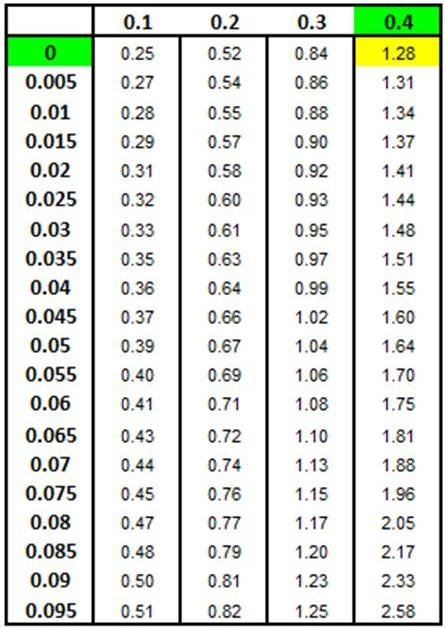Answer: The delivery time of 14.87 minutes is faster (smaller) than 90% of all delivery times.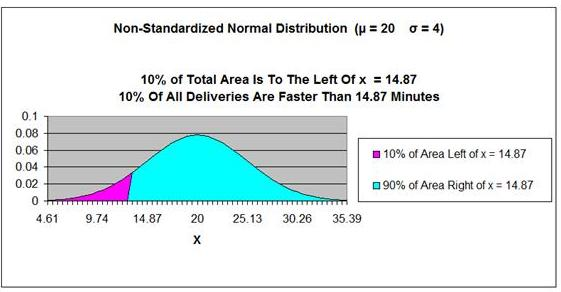This same problem is solved in the Excel Statistical Master with only 1 Excel formula (and not having to look up anything on a Z Chart - that's something you'll never have to do again with Excel). With the Excel Statistical Master you can do advanced business statistics without having to buy and learn expensive, complicated statistical software packages such as SyStat, MiniTab, SPSS, or SAS.

Problem 4

Problem 4: Using the Normal Distribution to Determine the Lower 10% Limit of Sales Bonuses

Salespeople of a large sales force received an annual bonus based upon performance. The size of the bonuses was Normally distributed with a mean of \$40,000 and a standard deviation of \$5000. What size bonus is exceeded by 90% of all other bonuses?

Problem Parameter Outline

Population Mean = µ = "mu" = \$40,000

Population Standard Deviation = σ = "sigma" =  \$5,000

x = ?

Probability that (Bonus ≥ x) = 90% = 0.90

Bonus is Normally distributed

Normal curve is not standardized (µ ≠ 0, σ ≠ 1)

Problem Solving Steps

We know that Bonus data is Normally distributed and can therefore be mapped on the Normal curve.

We are trying to determine Bonus amount will be lower than 90% of all other Bonuses.

This probability corresponds to the x value at which 90% of area under the Normal curve has a greater value and is to the right this x value. This x value must therefore be in the left tail of the Normal curve.

If we know that 90% of the area under the Normal curve is to the right of this x value (this is illustrated in the graph below), then we know that 40% of the total area under the Normal curve is between this x value and the mean. The remaining 50% of the area under the Normal curve makes up the half the Normal curve that is on the opposite side (the right side) of the mean.

If we know that 40% of the area under the Normal curve is between this x value and the mean, we can use the Z Score Chart to determine how many standard deviations this x value is from the mean. The Z Score Chart below shows this x value to be 1.28 standard deviations from the mean.

If we know how many standard deviations this x value is from the mean, we can use the following formula to calculate the x value, as follows:

z = ( x - µ ) / σ

x = z * σ + µ

x =  (-1.28) * 5,000 + 40,000  =  33,600

A Bonus of \$33,600 smaller than 90% of all Bonus amounts. This is illustrated in the graph below.

Answer: A Bonus of \$33,600 smaller than 90% of all Bonus amounts.

Z Score Chart

Z Score at x (Inner Numbers - Yellow)
vs.
Area Under Normal Curve
Between Mean (µ) and x  (Outer Numbers - Green)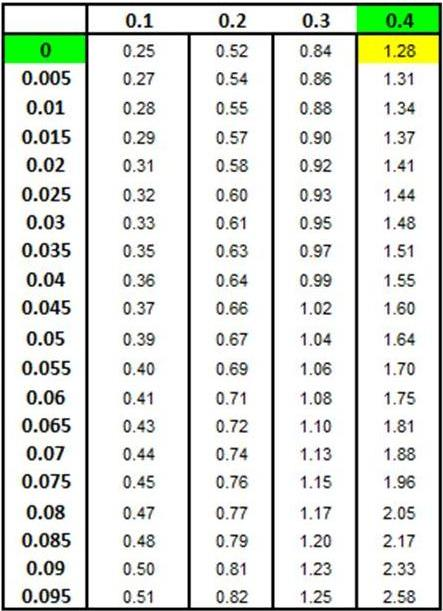Answer: A Bonus of \$33,600 is smaller than 90% of all Bonus amounts.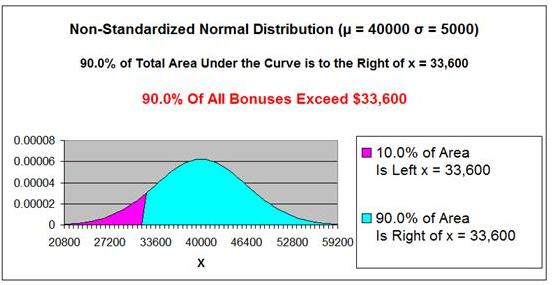This same problem is solved in the Excel Statistical Master with only 1 Excel formula (without having to look up anything on a Z Chart). If you found your statistics book confusing, You'll really like the Excel Statistical Master. Everything is explained in simple, step-by-step frameworks.

Problem 5

Problem 5: Using the Normal Distribution to Determine the Boundaries of the 75% Mid-Range of Sales Bonuses

Salespeople of a large sales force received an annual bonus based upon performance. The size of the bonuses was Normally distributed with a mean of \$40,000 and a standard deviation of \$5000. What would be the mid range that 75% of all bonuses would be in?

Problem Parameter Outline

Population Mean = µ = "mu" = \$40,000 miles

Population Standard Deviation = σ = "sigma" = \$5,000

x1 = ?   x2 = ?

Left Boundary
Probability that (Bonus ≤ x1 ) = 12.5% = 0.125

And

Right Boundary
Probability that (Bonus ≤ x2 ) = 87.5% = 0.875

Bonus is Normally distributed

Normal curve is not standardized (µ ≠ 0, σ ≠ 1)

Problem Solving Steps

We know that Bonus data is Normally distributed and can therefore be mapped on the Normal curve.

We are trying to determine the range of Bonuses that will be in the mid 75% of all Bonus amounts.12.5% of all Bonuses will lower than this mid-range and 12.5 of all Bonuses will be higher than this mid-range.

75% of all Bonuses corresponds to 75% of the area under this Normal curve.

x1 is the x value in which 12.5% of all Bonuses (and 12.5% of the area under the Normal curve) is less than. x1 exists in the outer left tail of the Normal curve. This can be observed in the graph below.

x2 is the x value in which 12.5% of all Bonuses (and 12.5% of the area under the Normal curve) is greater than than. x2 exists in the outer left tail of the Normal curve. This can be observed in the graph below.

Both x1 and x2 each have 12.5% of the total Normal curve area existing outside of their values. This means that 37.5% of the total area under the Normal curve exists the mean and each x value. This can be observed from the graph below.

The number of standard deviations between each x value and the mean can be found on a Z Chart. The Z value for the point that has 37.5% of the total curve area between it and the mean is 1.15. This can be observed in the Z Chart below. zx1 = -1.15 and zx2 = 1.15. Each of these x values is therefore 1.15 standard deviations from the mean but on opposite sides of the mean. This means that the two Z values will have opposite signs.

If we know how many standard deviations (the Z Scores) that these x value are from the mean, we can use the following formula to calculate the x values, as follows:

z = ( x - µ ) / σ

x = z * σ + µ

x1 =  (-1.15) * 5,000 + 40,000  =  34,250

x2 =  1.15 * 5,000 + 40,000  =  45,750

75% of all Bonuses fall between \$34,250 and \$45,750. This can be observed on the graph below.

Answer: 75% of all Bonuses fall between \$34,250 and \$45,750. This can be observed on the graph below.

Z Score Chart

Z Score at x (Inner Numbers - Yellow)
vs.
Area Under Normal Curve
Between Mean (µ) and x  (Outer Numbers - Green)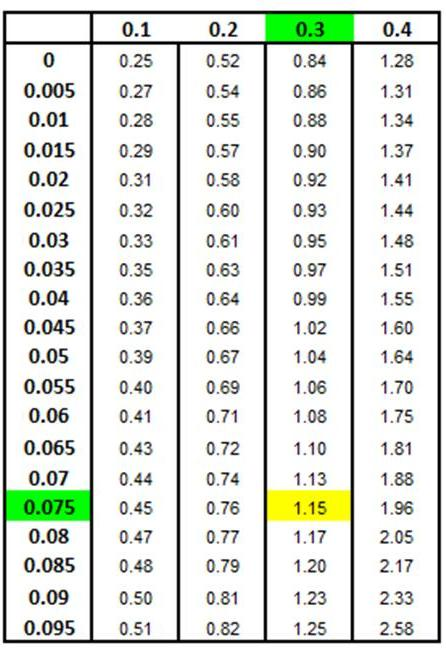Answer: 75% of all Bonuses fall between \$34,250 and \$45,750. This can be observed on the graph below.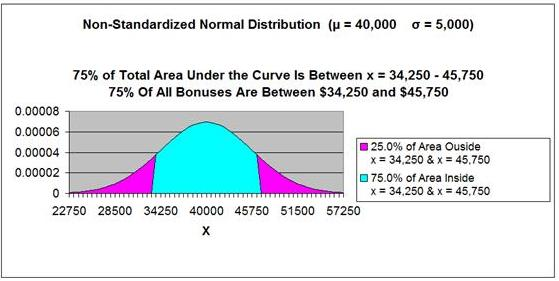This same problem is solved in the Excel Statistical Master with only 2 Excel formulas (and never again having to look up anything on a Z Chart). The Excel Statistical Master will make you a fully functional statistician at your workplace.

Problem 6

Problem 6: Using the Normal Distribution to Determine  the Probability that a Taxi Driver's Daily Mileage Will Be Within 1 of 2 Ranges

A large taxicab company analyzed the daily miles driven by each driver. The average distance was 200 miles with a standard deviation of 30 miles. A driver's mileage is selected at random. What is the probability that the this daily mileage will be either more than 261.5 mile OR less than 169.4 miles?

Problem Parameter Outline

Population Mean = µ = "mu" = 200 miles

Population Standard Deviation = σ = "sigma" = 30 miles

169.4 miles ≥ x

OR

x ≥ 261.5 miles

Probability that 169.4 miles ≥ x  OR  x ≥ 261.5 miles = ?

mileage is Normally distributed

Normal curve is not standardized (µ ≠ 0, σ ≠ 1)

Problem Solving Steps

We know that Daily Mileage data is Normally distributed and can therefore be mapped on the Normal curve.

We are trying to determine the probability that Daily Mileage will be either be greater than 261.4 OR less than 169.4 miles on any given day.

This probability corresponds to the percentage of area under the Normal curve outside of (greater than or to the right of) x1 = 261.5 PLUS the percentage of area under the Normal curve outside of (less than or to the left of) x2 = 169.4. This can be observed on the graph below.

We can calculate the Z values associated with each x with this formula as follows:

z = ( x - µ ) / σ

zx1 = ( 261.5 - 200 ) / 30 = 2.05

zx2 = ( 169.4 - 200 ) / 30 = -1.02

Because we know the Z values associated with each x value (zx1 and zx2), we can determine how much curve area exists between each x value and the mean.

The 1st Z chart below shows that 48% of the total area under the Normal curve exists between x1 and the mean. This can also be observed in the graph below.

The 2nd Z chart below shows that 34.5% of the total area under the Normal curve exists between x2 and the mean. This can also be observed in the graph below.

The total area under the Normal curve outside x = 261.5 and x = 169.4 equals the area outside of (to the right) of x = 261.5 (2% --->  50% - 48% = 2%) PLUS the area outside of (to the left of) x = 169.4 (15.5% ---> 50% - 34.5% = 15.5%).

2% + 15.5% = 17.5%

17.5% of the total area under the Normal curve exists outside of x = 261.5 and x = 169.4. This is shown in the graph below.

Answer: There is a 17.5% probability that a taxi driver's Daily Mileage will be greater than 261.5 or less than 169.4 miles on any given day.

Z Score Chart

Z Score at x (Inner Numbers - Yellow)
vs.
Area Under Normal Curve
Between Mean (µ) and x  (Outer Numbers - Green)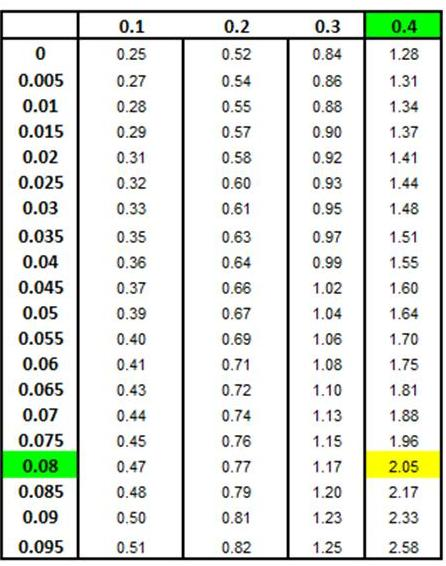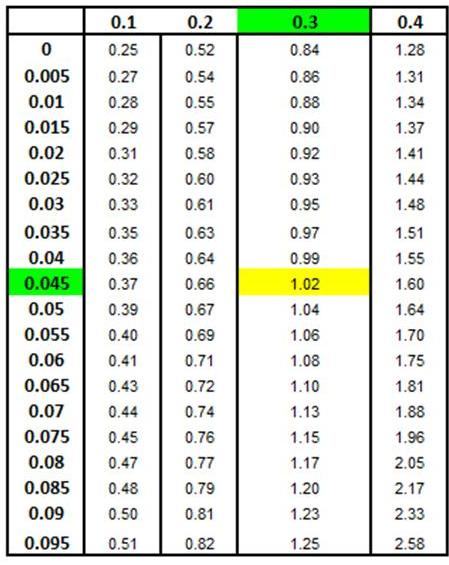Answer: There is a 17.5% probability that a taxi driver's Daily Mileage will be greater than 261.5 or less than 169.4 miles on any given day.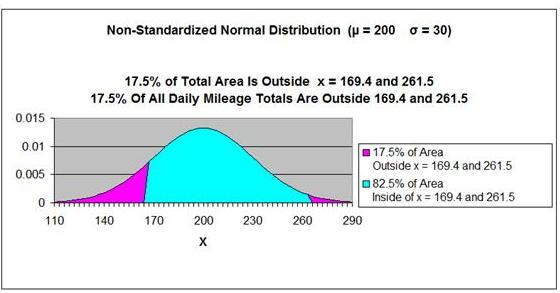This same problem is solved in the Excel Statistical Master with only 2 Excel formulas. The Excel Statistical Master is the fastest way for you to climb the business statistics learning curve.

Interesting Bit of Trivia